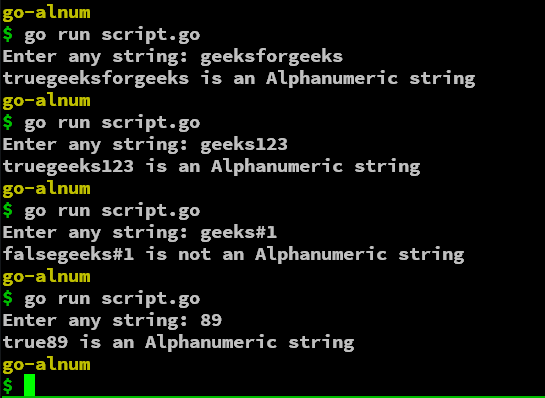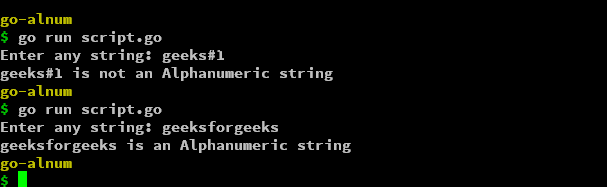# Golang Program to Check if the String is Alphanumeric

• Last Updated : 04 Apr, 2022

In this article, we will see how to validate an alphanumeric string in Golang. We will simply input a string from the user and check if the string is alphanumeric or not.  We will be using the regexp module in Go to validate the alphanumeric patterning of the string.

### String Input

To get the input from the user, we will be using the Scan function from the fmt module in Golang. We will store the input in a string variable.

## Go

 `// Golang program to take input string from user` `package` `main`   `import` `(` `    ``"fmt"` `)`   `func` `main() {` `    ``var` `word ``string` `    ``fmt.Print(``"Enter any string: "``)` `    ``fmt.Scan(&word)` `}`

In the above program, we have initialized a variable of type string. Followed by declaration we have a simple text message for the user input. Finally, we use the Scan function and store the input in the variable.

### Checking For Alphanumeric Regex

After we have the input from the user, we can now move towards validating the string as an alphanumeric string. To do that, we will first import the regexp module. After importing the module, we will access to MustCompile and MatchString functions.

## Go

 `// Go program to check Alphanumeric Regex` `package` `main`   `import` `(` `    ``"fmt"` `    ``"regexp"` `)`   `func` `main() {` `    ``var` `word ``string` `    ``fmt.Print(``"Enter any string: "``)` `    ``fmt.Scan(&word)` `    ``is_alphanumeric := regexp.MustCompile(`^[a-zA-Z0-``9``]*\$`).MatchString(word)` `}`

Using the MustCompiler function, we can check if the regular expression is satisfied or not, We have parsed the string ^[a-zA-Z0-9_]*\$ which will check from start(^) to end(\$) any occurrences of the characters from 0-9, A-Z and a-z. We combine the function with MatchString which will compare the regular expression to the passed string. It will simply return true if the regular expression evaluated is matched with the string else false if the pattern is not matched.

Thus, by using the MustCompile and MatchString functions, we can validate any string to check if it’s alphanumeric or not. So, we can further use conditional statements to print the message accordingly.

## Go

 `// Go program to check Alphanumeric Regex` `package` `main`   `import` `(` `    ``"fmt"` `    ``"regexp"` `)`   `func` `main() {` `    ``var` `word ``string` `    ``fmt.Print(``"Enter any string: "``)` `    ``fmt.Scan(&word)` `    ``is_alphanumeric := regexp.MustCompile(`^[a-zA-Z0-``9``]*\$`).MatchString(word)` `    ``fmt.Print(is_alphanumeric)` `    ``if` `is_alphanumeric{` `        ``fmt.Printf(``"%s is an Alphanumeric string"``, word)` `    ``} ``else``{` `        ``fmt.Printf(``"%s is not an Alphanumeric string"``, word)` `    ``}` `}`

Output: So, the script is working as expected and giving the appropriate output for different string combinations.### Converting the Script into a function

We can convert the above script into a function for better usage irrespective of the requirement and conditions for the project.

## Go

 `// Go program to check Alphanumeric string` `package` `main`   `import` `(` `    ``"fmt"` `    ``"regexp"` `)`   `func` `is_alphanum(word ``string``) bool {` `    ``return` `regexp.MustCompile(`^[a-zA-Z0-``9``]*\$`).MatchString(word)` `}`   `func` `main() {` `    ``var` `word ``string` `    ``fmt.Print(``"Enter any string: "``)` `    ``fmt.Scan(&word)` `    ``is_alphanumeric := is_alphanum(word)` `    ``if` `is_alphanumeric{` `        ``fmt.Printf(``"%s is an Alphanumeric string"``, word)` `    ``} ``else``{` `        ``fmt.Printf(``"%s is not an Alphanumeric string"``, word)` `    ``}` `}`

Output:My Personal Notes arrow_drop_up
Recommended Articles
Page :